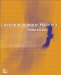# A.4 Sets

## A.4 Sets

A collection, class, or listing of mathematical objects is called a set. The objects in the set are referred to as elements or members. The universal set is the complete set of objects applicable to the object context (for example, if we were interested in performing operations on sets of prime numbers between 0 and 20, then the universal set would be {2, 3, 5, 7, 11, 13, 17, 19}). For statistical applications we are largely interested in sets of numbers; however, in areas of design such as topology analysis we may wish to manipulate a set of objects such as edges or adjacencies (described in Chapters 4 and 6). A set can be described using precise mathematical notation. Once described, there are algebraic operations that can be performed on sets using additional notation.

 {a,b,c,d,e, ... z} the set of elements from a to z φ the empty set A' the complement of set A (i.e., A becomes the universal set minus the intersection of set A and the universal set) n(A) the number of elements in set A ⋃ union of two sets (i.e., those elements common or unique to both sets) ⋂ intersection of two sets (i.e., those elements common to both sets) ∈ is a member/element of (e.g., 3 ∊ {1, 2, 3}). ∉ is not a member/element of (e.g., 3 ∉ {1, 2, 4, 8}). ⊆ is a subset of (e.g., if every element of set T is a member of set S, then T ⊆ S). ⊂ is a proper subset of (e.g., T ⊂ S in the above example, if an element s ∊ S, and s ∉ T).

For example: the set containing 0 and 1 is denoted as {0, 1}. The set of even numbers between 2 and 500 is denoted as {2, 4, 6, ..., 500}. Regarding subsets, set S = {2, 4, 6} has 8 distinct subsets: {2, 4, 6}, {2}, {4}, {6}, {2, 4}, {2, 6}, {4, 6}, plus the empty set {}, denoted as φ.ActionScripting in Flash MX
ISBN: N/A
EAN: 2147483647
Year: 2001
Pages: 117